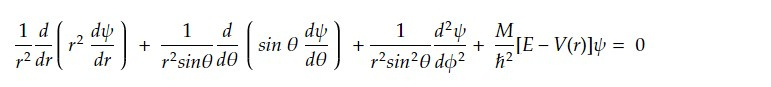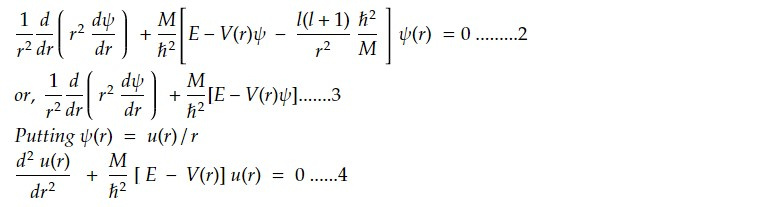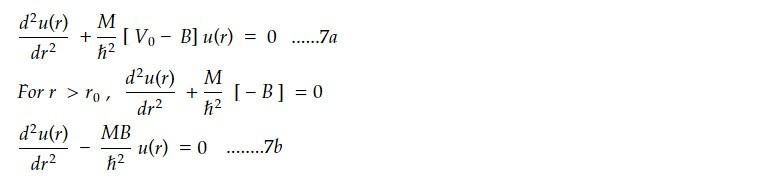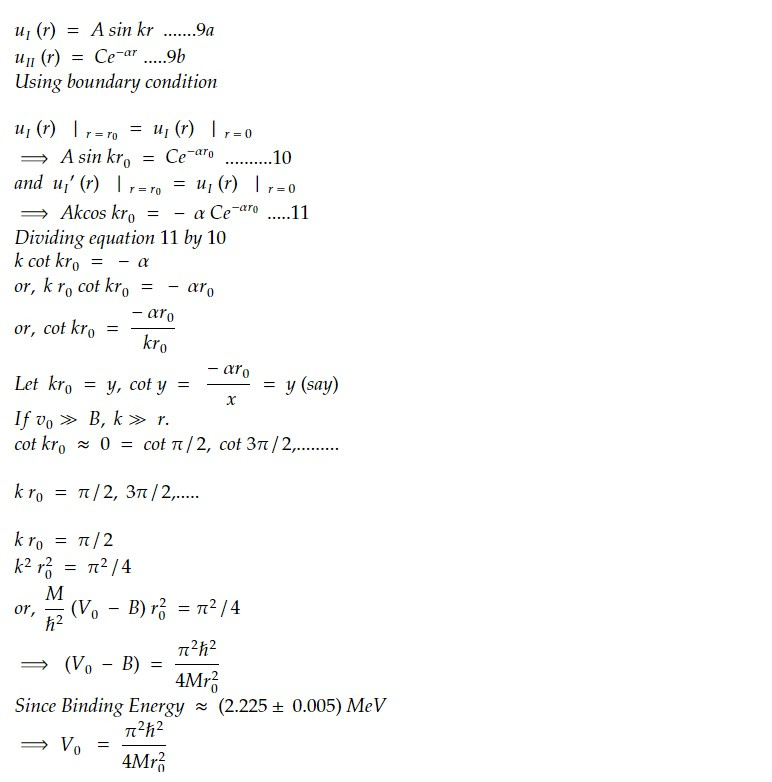# Deuteron problem

The deuteron is also important for understanding the behaviour of nuclear forces, which are responsible for holding atomic nuclei together.

The solution of the deuteron problem paved the way for the development of nuclear physics and the study of nuclear reactions, which are important in a variety of fields, including nuclear energy, nuclear medicine, and astrophysics. The study of the deuteron and other atomic nuclei also provides insights into the structure and properties of matter at the subatomic level, and contributes to our understanding of the nature of the universe.

Deuteron nucleus consists of proton and a neutron and it remains mostly in ground state with zero orbital angular momentum (l =o).

The ground state of deuterium is the lowest energy state of the deuteron, which is the bound state of a proton and a neutron. The ground state of deuterium has a total spin of 1 and a positive parity.

The energy of the ground state of deuterium is -2.225 MeV, which is the binding energy of the deuteron. This energy corresponds to the amount of energy that is required to break the deuteron into its constituent proton and neutron.

The wave function of the ground state of deuterium is a combination of the spatial wave function and the spin wave function. The spatial wave function describes the probability density of finding the deuteron at a given position, while the spin wave function describes the spin orientation of the deuteron.

The ground state of deuterium has a spatial wave function that is spherically symmetric, since the deuteron is a spin-1 particle and does not have any intrinsic angular momentum. The spin wave function of the ground state of deuterium is antisymmetric, which means that it changes sign when the positions of the proton and neutron are interchanged.

The ground state of deuterium is important for understanding the properties of atomic nuclei, and provides a starting point for studying the excited states of the deuteron and other atomic nuclei. The ground state of deuterium is also important for understanding the behaviour of nuclear forces and for developing models of nuclear structure and reactions.

Let V= V(r) be central potential that describes deuteron in its ground where 'r' be relative separation between two nucleons. Schrodinger equation for two body problem is given byIn Spherical polar coordinate system equation 1 isTaking radial path only and putting l=0 for ground state deuteronLet us approximate the central potential V(r) by a squared well potential and defined asFor the bound system, total energy E is negative. Therefore

E = - B = -2.226 MeV ........6

From equation 6 and 5, equation 4 becomes,Solution of 7a and 7b areUsual condition for wavefunction 𝜓(r) are 𝜓(r) -> finite at r-> 0 and 𝜓(r) -> 0 at r-> ∞.

Thus u(r) = r 𝜓(r) -> 0 as r -> 0. This condition demands B'=0 is 8a. Similarly, u(r) = r 𝜓(r) -> 0 as r -> ∞. This condition demands D = 0 in 8b.

Equation 8a and 8b becomesThis note is a part of the Physics Repository.